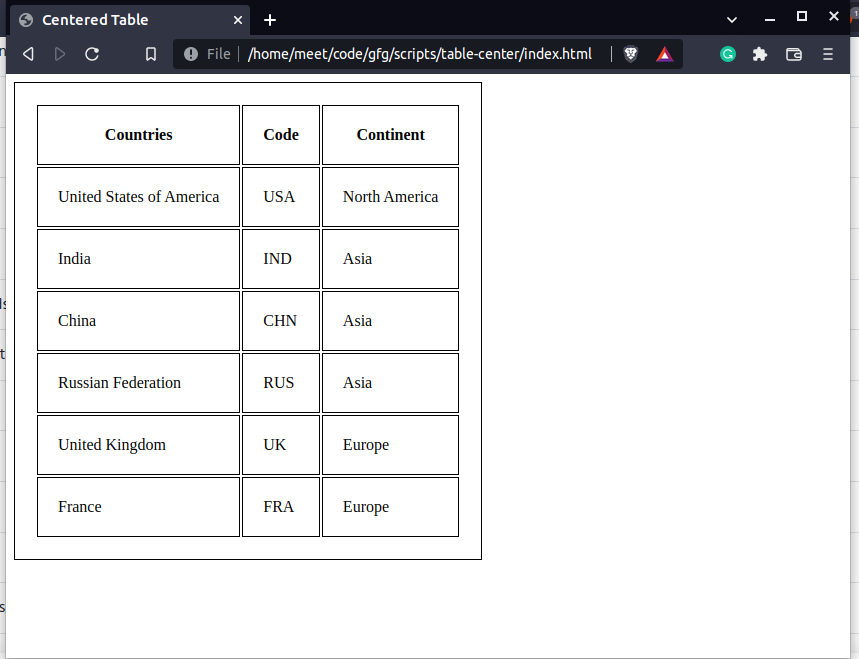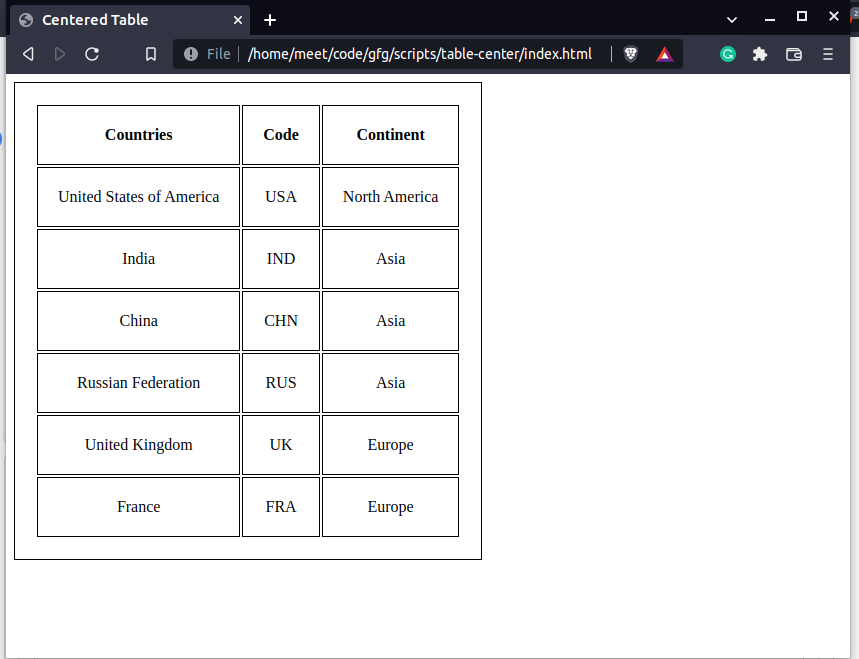GeeksforGeeks App
Open AppBrowser
Continue

# How to center contents of an HTML table ?

In this article, we will be demonstrating how to align the contents of a table in the center. The text-align property in CSS can be used to align the contents of the given container or an element.

Approach: To align the contents of a table in the center, we can use the text-align property in CSS. The element table can be styled using the text-align property and the value as center will result in the desired solution. By default the text-align property is set to left, so the contents are aligned to the left, we will override the value of this property to center to align the contents as per our requirement.

Syntax:

`text-align: left;`

Property Value:

• initial: It is the default value.
• inherit: It sets the value to the parent’s element property value.
• left: It align the text to left (default property).
• right: It align the text to the right.
• center: It align the text in the center.
• justify: It stretches the content of an element.

Example 1: We will first create an HTML template with the table element and add the data to the table rows and columns.

## HTML

 ```<``html` `lang``=``"en"``>` `<``head``>``    ``<``meta` `charset``=``"UTF-8"``>``    ``<``title``>Centered Table``    ``<``style``>``        ``table,``        ``td,``        ``th {``            ``border: 1px solid;``            ``padding: 20px;``        ``}``    ````` `<``body``>``    ``<``table``>``        ``<``tr``>``            ``<``th``>Countries``            ``<``th``>Code``            ``<``th``>Continent``        ````        ``<``tr``>``            ``<``td``>United States of America``            ``<``td``>USA``            ``<``td``>North America``        ````        ``<``tr``>``            ``<``td``>India``            ``<``td``>IND``            ``<``td``>Asia``        ````        ``<``tr``>``            ``<``td``>China``            ``<``td``>CHN``            ``<``td``>Asia``        ````        ``<``tr``>``            ``<``td``>Russian Federation``            ``<``td``>RUS``            ``<``td``>Asia``        ````        ``<``tr``>``            ``<``td``>United Kingdom``            ``<``td``>UK``            ``<``td``>Europe``        ````        ``<``tr``>``            ``<``td``>France``            ``<``td``>FRA``            ``<``td``>Europe``        ````    ````` ``

Output:Here, we can see that the table cell elements are aligned to the left by default. We have applied some basic styles like border and padding to make the table a bit more representable. These styles like the border have been set for visualizing the alignment with the border and also adding padding for scaling in the table view a bit more and being able to see the align property in a better way.

Aligning the contents in the center: Now, since we have added the contents of the table, we can add a CSS property to align the contents of the table to the center. We can use the table element and add the property of text-align by setting it to the center. You have to add the following code in the style tag as below:

```<style>
table,
td,
th {
border: 1px solid;
}

table {
text-align: center;
}
</style>```

Example 2: This example will demonstrate the text of the table in the center using CSS.

## HTML

 ```<``html` `lang``=``"en"``>` `<``head``>``    ``<``meta` `charset``=``"UTF-8"``>``    ``<``title``>Centered Table``    ``<``style``>``        ``table,``        ``td,``        ``th {``            ``border: 1px solid;``            ``padding: 20px;``        ``}` `        ``table {``            ``text-align: center;``        ``}``    ````` `<``body``>``    ``<``table``>``        ``<``tr``>``            ``<``th``>Countries``            ``<``th``>Code``            ``<``th``>Continent``        ````        ``<``tr``>``            ``<``td``>United States of America``            ``<``td``>USA``            ``<``td``>North America``        ````        ``<``tr``>``            ``<``td``>India``            ``<``td``>IND``            ``<``td``>Asia``        ````        ``<``tr``>``            ``<``td``>China``            ``<``td``>CHN``            ``<``td``>Asia``        ````        ``<``tr``>``            ``<``td``>Russian Federation``            ``<``td``>RUS``            ``<``td``>Asia``        ````        ``<``tr``>``            ``<``td``>United Kingdom``            ``<``td``>UK``            ``<``td``>Europe``        ````        ``<``tr``>``            ``<``td``>France``            ``<``td``>FRA``            ``<``td``>Europe``        ````    ````` ``

Output:So by using the text-align property for the table element,  we can center the contents of the table.  The contents of the table are aligned in the center depending on the amount of content the table cell has, if it is small enough to fit in the center, it is not clearly seen how the contents are aligned. Also, if the contents of the table cells are big enough they are aligned in the center and it becomes noticeable.

So, this is how we align the contents of an HTML table in the center using CSS.

My Personal Notes arrow_drop_up
Related Tutorials